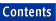Apache C++ Standard Library Reference Guide

## istream_iterator

Library:  Iterators

`istream_iteratoriterator`

### Summary

A stream iterator that has iterator capabilities for istreams. This iterator allows generic algorithms to be used directly on streams.

### Synopsis

```#include <iterator>

namespace std {
template <class T, class charT,
class traits = char_traits<charT>,
class Distance = ptrdiff_t>
class istream_iterator :
public iterator<input_iterator_tag, T,
Distance, const T*, const T&>;
}
```

### Description

Stream iterators are the standard iterator interface for input and output streams.

The class template istream_iterator reads elements from an input stream using operator >>(). A value of type T is retrieved and stored when the iterator is constructed and each time operator++() is called. The iterator is equal to the end-of-stream iterator value if the end-of-file is reached. You can use the constructor with no arguments to create an end-of-stream iterator. The only valid use of this iterator is to compare to other iterators when checking for end of file. Do not attempt to dereference the end-of-stream iterator; it plays the same role as the past-the-end iterator of the end() function of containers. Since an istream_iterator is an input iterator, you cannot assign to the value returned by dereferencing the iterator. This also means that istream_iterators can only be used for single pass algorithms.

Since a new value is read every time the operator++() is used on an istream_iterator, that operation is not equality-preserving. This means that i == j does not mean that ++i == ++j (although two end-of-stream iterators are always equal).

### Interface

```namespace std {
template <class T, class charT,
class traits = char_traits<charT>,
class Distance = ptrdiff_t>
class istream_iterator :
public iterator<input_iterator_tag, T, Distance,
const T*, const T&>
{

public:
typedef T value_type;
typedef charT char_type;
typedef traits traits_type;
typedef basic_istream<charT,traits> istream_type;

istream_iterator();
istream_iterator(istream_type&);
istream_iterator(const stream_iterator&);

const T& operator*() const;
const T* operator->() const;
istream_iterator& operator++();
istream_iterator  operator++(int);
};

// Nonmember Operators

template <class T, class charT, class traits,class Distance>
bool operator==(const istream_iterator<T,charT,traits,Distance>&,
const istream_iterator<T,charT,traits,Distance>&);

template <class T, class charT, class traits,class Distance>
bool operator!=(const istream_iterator<T,charT,traits,Distance>&,
const istream_iterator<T,charT,traits,Distance>&);
}
```

### Member Types

`char_type; `

Type of character the stream is built on.

`traits_type; `

Traits used to build the stream.

`istream_type; `

Type of stream this iterator is constructed on.

### Constructors

`istream_iterator();`

Constructs an end-of-stream iterator. This iterator can be used to compare against an end-of-stream condition. Use it to provide end iterators to algorithms.

`istream_iterator(istream_type& s);`

Constructs an istream_iterator on the given stream.

`istream_iterator(const istream_iterator& x);`

Copy constructor.

### Operators

```const T&
operator*() const;```

Returns the current value stored by the iterator.

```const T*
operator->() const;```

Returns a pointer to the current value stored by the iterator.

```istream_iterator& operator++()
istream_iterator operator++(int)```

Retrieves the next element from the input stream.

### Nonmember Operators

```template <class T, class charT, class traits, class Distance>
bool
operator==(const istream_iterator<T,charT,traits,
Distance>& x,
const
istream_iterator<T,charT,traits,Distance>& y)```

Returns true if x and y both refer to the same stream or are both past-the-end.

```template <class T, class charT, class traits, class Distance>
bool
operator!=(const istream_iterator<T,charT,traits,Distance>& x,
const istream_iterator<T,charT,traits,Distance>& y)```

Returns !(x == y).

### Example

```//
//  io_iter.cpp
//

#include <algorithm>  // for copy
#include <iostream>   // for cin, cout, endl
#include <iterator>   // for stream_iterators, inserter
#include <vector>     // for vector
#include <numeric>    // for accumulate

int main ()
{
// Typedefs for convenience.
typedef std::vector<int, std::allocator<int> >    Vector;

typedef std::istream_iterator<Vector::value_type,
char, std::char_traits<char>,
ptrdiff_t>                   is_iter;

typedef std::ostream_iterator<Vector::value_type, char,
std::char_traits<char> >     os_iter;

Vector v;

Vector::value_type sum = 0;

// Collect values from cin until end of file
// Note use of default constructor to get ending iterator
std::cout << "Enter a sequence of integers (eof to quit): ";
std::copy (is_iter (std::cin), is_iter (),
std::inserter (v, v.begin ()));

// Stream the whole vector and the sum to cout.
std::copy (v.begin (), v.end () -1,
os_iter (std::cout, " + "));

if (v.size ())
std::cout << v.back () << " = "
<< std::accumulate (v.begin (), v.end (), sum)
<< std::endl;

return 0;
}

Program Output:
```
```Enter a sequence of integers (eof to quit): 1 + 1 + 2 + 3 + 5 +
8 + 13 + 21 + 45 + 66 + 111 + 177 = 453
```